CHIRAG GOYAL — Published On May 20, 2021 and Last Modified On July 6th, 2021

This article was published as a part of the Data Science Blogathon

## Introduction

Building even a simple neural network can be a confusing task and tuning it to get the better result is an extremely tedious task. The most common problem with Deep Neural Networks is Vanishing and Exploding gradient descent. To solve these issues, one solution could be to initialize the parameters carefully. In this article, we will discuss Weight initialization techniques.

This article has been written under the assumption that you have a basic understanding of neural networks, weights, biases, activation functions, forward and backward propagation, etc.

• 👉 Basics and notations of neural networks
• 👉 Steps of training a neural network
• 👉 Why weight initialization?
• 👉 Different Weight initialization techniques
• 👉 Best practices of weight initialization
• 👉 Conclusion

## Basics and Notations

Consider a neural network having an l layer, which has l-1 hidden layers and 1 output layer. Then, the parameters i.e, weights and biases of the layer l are represented as,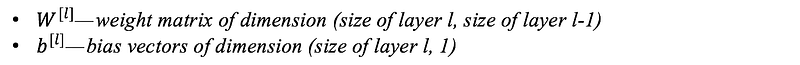Image Source:

In addition to weights and biases, some more intermediate variables are also computed during the training process,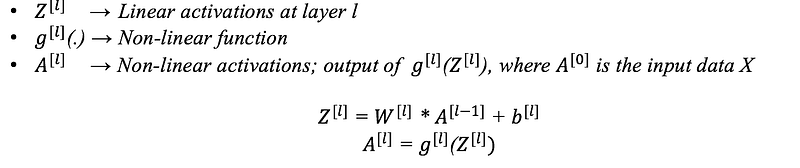Image Source:

## Steps of Training a Neural Network

Training a neural network consists of the following basic steps:

Step-1: Initialization of Neural Network: Initialize weights and biases.

Step-2: Forward propagation: Using the given input X, weights W, and biases b, for every layer we compute a linear combination of inputs and weights (Z)and then apply activation function to linear combination (A). At the final layer, we compute f(A(l-1)) which could be a sigmoid (for binary classification problem), softmax (for multi-class classification problem), and this gives the prediction y_hat.

Step-3: Compute the loss function: The loss function includes both the actual label y and predicted label y_hat in its expression. It shows how far our predictions from the actual target, and our main objective is to minimize the loss function.

Step-4: Backward Propagation: In backpropagation, we find the gradients of the loss function, which is a function of y and y_hat, and gradients wrt A, W, and b called dA, dW, and db. By using these gradients, we update the values of the parameters from the last layer to the first layer.

Step-5: Repeat steps 2–4 for n epochs till we observe that the loss function is minimized, without overfitting the train data.

For Example,

For a neural network having 2 layers, i.e. one hidden layer. (Here bias term is not added just for the simplicity)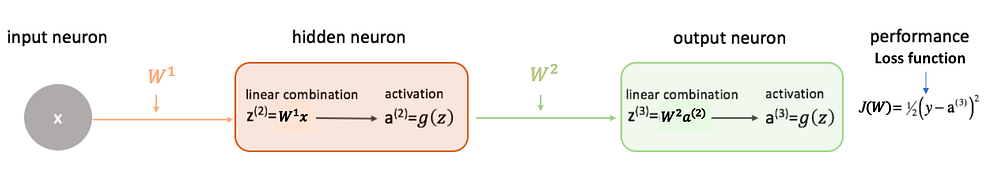Fig. Forward Propagation

Image Source: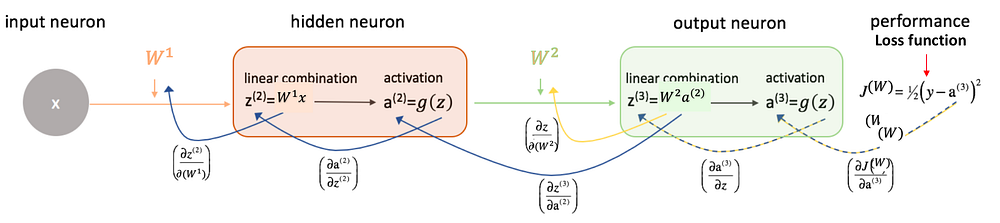Fig. Backward Propagation

Image Source:

## Why Weight Initialization?

Its main objective is to prevent layer activation outputs from exploding or vanishing gradients during the forward propagation. If either of the problems occurs, loss gradients will either be too large or too small, and the network will take more time to converge if it is even able to do so at all.

If we initialized the weights correctly, then our objective i.e, optimization of loss function will be achieved in the least time otherwise converging to a minimum using gradient descent will be impossible.

## Different Weight Initialization Techniques

One of the important things which we have to keep in mind while building your neural network is to initialize your weight matrix for different connections between layers correctly.

Let us see the following two initialization scenarios which can cause issues while we training the model:

### Zero Initialization (Initialized all weights to 0)

If we initialized all the weights with 0, then what happens is that the derivative wrt loss function is the same for every weight in W[l], thus all weights have the same value in subsequent iterations. This makes hidden layers symmetric and this process continues for all the n iterations. Thus initialized weights with zero make your network no better than a linear model. It is important to note that setting biases to 0 will not create any problems as non-zero weights take care of breaking the symmetry and even if bias is 0, the values in every neuron will still be different.

### Random Initialization (Initialized weights randomly)

– This technique tries to address the problems of zero initialization since it prevents neurons from learning the same features of their inputs since our goal is to make each neuron learn different functions of its input and this technique gives much better accuracy than zero initialization.

– In general, it is used to break the symmetry. It is better to assign random values except 0 to weights.

– Remember, neural networks are very sensitive and prone to overfitting as it quickly memorizes the training data.

Now, after reading this technique a new question comes to mind: “What happens if the weights initialized randomly can be very high or very low?”

•  For any activation function, abs(dW) will get smaller and smaller as we go backward with every layer during backpropagation especially in the case of deep neural networks. So, in this case, the earlier layers’ weights are adjusted slowly.
• Due to this, the weight update is minor which results in slower convergence.
• This makes the optimization of our loss function slow. It might be possible in the worst case, this may completely stop the neural network from training further.
• More specifically, in the case of the sigmoid and tanh and activation functions, if your weights are very large, then the gradient will be vanishingly small, effectively preventing the weights from changing their value. This is because abs(dW) will increase very slightly or possibly get smaller and smaller after the completion of every iteration.
• So, here comes the use of the RELU activation function in which vanishing gradients are generally not a problem as the gradient is 0 for negative (and zero) values of inputs and 1 for positive values of inputs.

• This is the exact opposite case of the vanishing gradients, which we discussed above.
• Consider we have weights that are non-negative, large, and having small activations A. When these weights are multiplied along with the different layers, they cause a very large change in the value of the overall gradient (cost). This means that the changes in W, given by the equation W= W — ⍺ * dW, will be in huge steps, the downward moment will increase.

Problems occurred due to exploding gradients:

– This problem might result in the oscillation of the optimizer around the minima or even overshooting the optimum again and again and the model will never learn!

– Due to the large values of the gradients, it may cause numbers to overflow which results in incorrect computations or introductions of NaN’s (missing values).

## Best Practices for Weight Initialization

👉 Use RELU or leaky RELU as the activation function, as they both are relatively robust to the vanishing or exploding gradient problems (especially for networks that are not too deep). In the case of leaky RELU, they never have zero gradients. Thus they never die and training continues.

👉 Use Heuristics for weight initialization: For deep neural networks, we can use any of the following heuristics to initialize the weights depending on the chosen non-linear activation function.

While these heuristics do not completely solve the exploding or vanishing gradients problems, they help to reduce it to a great extent. The most common heuristics are as follows:

(a) For RELU activation function: This heuristic is called He-et-al Initialization.

In this heuristic, we multiplied the randomly generated values of W by: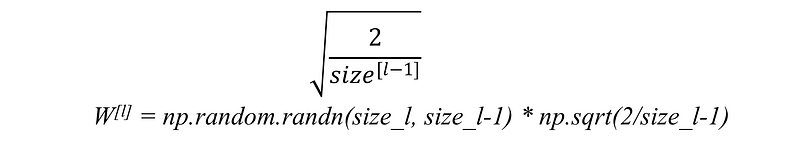Image Source:

(b) For tanh activation function : This heuristic is known as Xavier initialization.

In this heuristic, we multiplied the randomly generated values of W by: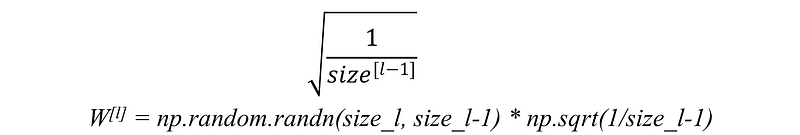Image Source:

(c) Another commonly used heuristic is: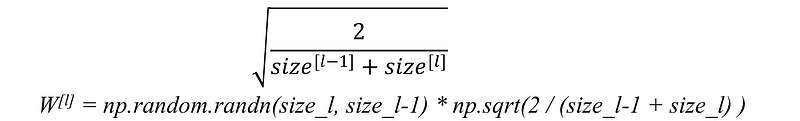Image Source:

Benefits of using these heuristics:

• All these heuristics serve as good starting points for weight initialization and they reduce the chances of exploding or vanishing gradients.
• All these heuristics do not vanish or explode too quickly, as the weights are neither too much bigger than 1 nor too much less than 1.
• They help to avoid slow convergence and ensure that we do not keep oscillating off the minima.

👉 Gradient Clipping:  It is another way for dealing with the exploding gradient problem. In this technique, we set a threshold value, and if our chosen function of a gradient is larger than this threshold, then we set it to another value.

NOTE: In this article, we have talked about various initializations of weights, but not the biases since gradients wrt bias will depend only on the linear activation of that layer, but not depend on the gradients of the deeper layers. Thus, there is not a problem of diminishing or explosion of gradients for the bias terms. So, Biases can be safely initialized to 0.

## Conclusion

👉 Zero initialization causes the neuron to memorize the same functions almost in each iteration.

👉 Random initialization is a better choice to break the symmetry. However, initializing weight with much high or low value can result in slower optimization.

👉 Using an extra scaling factor in Xavier initialization, He-et-al Initialization, etc can solve the above issue to some extent. That’s why these are the more recommended weight initialization methods among all.

## End Notes

If you liked this and want to know more, go visit my other articles on Data Science and Machine Learning by clicking on the Link

Something not mentioned or want to share your thoughts? Feel free to comment below And I’ll get back to you.

## Chirag Goyal

Currently, I am pursuing my Bachelor of Technology (B.Tech) in Computer Science and Engineering from the Indian Institute of Technology Jodhpur(IITJ). I am very enthusiastic about Machine learning, Deep Learning, and Artificial Intelligence.

The media shown in this article are not owned by Analytics Vidhya and is used at the Author’s discretion.•